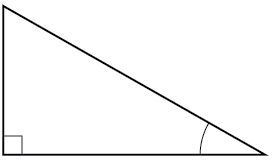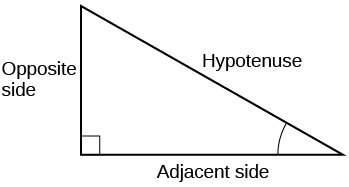7.2 Right triangle trigonometry  (Page 5/12)

 Page 5 / 12

How long a ladder is needed to reach a windowsill 50 feet above the ground if the ladder rests against the building making an angle of $\text{\hspace{0.17em}}\frac{5\pi }{12}\text{\hspace{0.17em}}$ with the ground? Round to the nearest foot.

Access these online resources for additional instruction and practice with right triangle trigonometry.

Key equations

 Trigonometric Functions Reciprocal Trigonometric Functions Cofunction Identities

Key concepts

• We can define trigonometric functions as ratios of the side lengths of a right triangle. See [link] .
• The same side lengths can be used to evaluate the trigonometric functions of either acute angle in a right triangle. See [link] .
• We can evaluate the trigonometric functions of special angles, knowing the side lengths of the triangles in which they occur. See [link] .
• Any two complementary angles could be the two acute angles of a right triangle.
• If two angles are complementary, the cofunction identities state that the sine of one equals the cosine of the other and vice versa. See [link] .
• We can use trigonometric functions of an angle to find unknown side lengths.
• Select the trigonometric function representing the ratio of the unknown side to the known side. See [link] .
• Right-triangle trigonometry facilitates the measurement of inaccessible heights and distances.
• The unknown height or distance can be found by creating a right triangle in which the unknown height or distance is one of the sides, and another side and angle are known. See [link] .

Verbal

For the given right triangle, label the adjacent side, opposite side, and hypotenuse for the indicated angle.When a right triangle with a hypotenuse of 1 is placed in a circle of radius 1, which sides of the triangle correspond to the x - and y -coordinates?

The tangent of an angle compares which sides of the right triangle?

The tangent of an angle is the ratio of the opposite side to the adjacent side.

What is the relationship between the two acute angles in a right triangle?

Explain the cofunction identity.

For example, the sine of an angle is equal to the cosine of its complement; the cosine of an angle is equal to the sine of its complement.

Algebraic

For the following exercises, use cofunctions of complementary angles.

$\mathrm{cos}\left(34°\right)=\mathrm{sin}\left(___°\right)$

$\mathrm{cos}\left(\frac{\pi }{3}\right)=\mathrm{sin}\left(___\right)$

$\frac{\pi }{6}$

$\mathrm{csc}\left(21°\right)=\mathrm{sec}\left(___°\right)$

$\mathrm{tan}\left(\frac{\pi }{4}\right)=\mathrm{cot}\left(___\right)$

$\frac{\pi }{4}$

For the following exercises, find the lengths of the missing sides if side $\text{\hspace{0.17em}}a\text{\hspace{0.17em}}$ is opposite angle $\text{\hspace{0.17em}}A,$ side $\text{\hspace{0.17em}}b\text{\hspace{0.17em}}$ is opposite angle $\text{\hspace{0.17em}}B,$ and side $\text{\hspace{0.17em}}c\text{\hspace{0.17em}}$ is the hypotenuse.

$\mathrm{cos}\text{\hspace{0.17em}}B=\frac{4}{5},a=10$

$\mathrm{sin}\text{\hspace{0.17em}}B=\frac{1}{2},a=20$

$b=\frac{20\sqrt{3}}{3},c=\frac{40\sqrt{3}}{3}$

$\mathrm{tan}\text{\hspace{0.17em}}A=\frac{5}{12},b=6$

$\mathrm{tan}\text{\hspace{0.17em}}A=100,b=100$

$a=10,000,c=10,00.5$

A laser rangefinder is locked on a comet approaching Earth. The distance g(x), in kilometers, of the comet after x days, for x in the interval 0 to 30 days, is given by g(x)=250,000csc(π30x). Graph g(x) on the interval [0, 35]. Evaluate g(5)  and interpret the information. What is the minimum distance between the comet and Earth? When does this occur? To which constant in the equation does this correspond? Find and discuss the meaning of any vertical asymptotes.
The sequence is {1,-1,1-1.....} has
how can we solve this problem
Sin(A+B) = sinBcosA+cosBsinA
Prove it
Eseka
Eseka
hi
Joel
June needs 45 gallons of punch. 2 different coolers. Bigger cooler is 5 times as large as smaller cooler. How many gallons in each cooler?
7.5 and 37.5
Nando
find the sum of 28th term of the AP 3+10+17+---------
I think you should say "28 terms" instead of "28th term"
Vedant
the 28th term is 175
Nando
192
Kenneth
if sequence sn is a such that sn>0 for all n and lim sn=0than prove that lim (s1 s2............ sn) ke hole power n =n
write down the polynomial function with root 1/3,2,-3 with solution
if A and B are subspaces of V prove that (A+B)/B=A/(A-B)
write down the value of each of the following in surd form a)cos(-65°) b)sin(-180°)c)tan(225°)d)tan(135°)
Prove that (sinA/1-cosA - 1-cosA/sinA) (cosA/1-sinA - 1-sinA/cosA) = 4
what is the answer to dividing negative index
In a triangle ABC prove that. (b+c)cosA+(c+a)cosB+(a+b)cisC=a+b+c.
give me the waec 2019 questionsByByBy RhodesBy Prateek AshtikarByBy